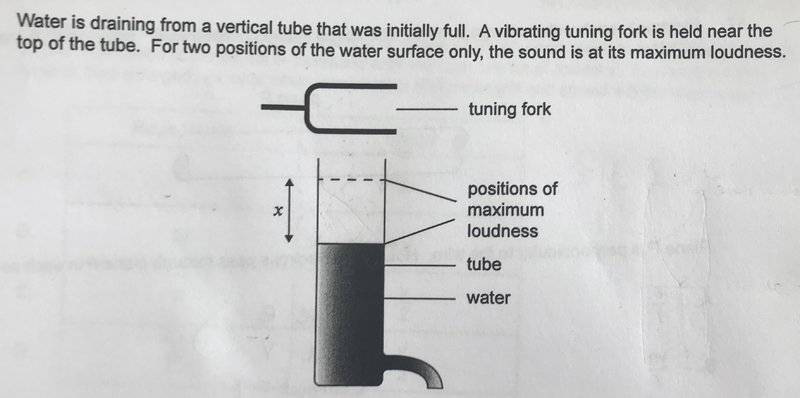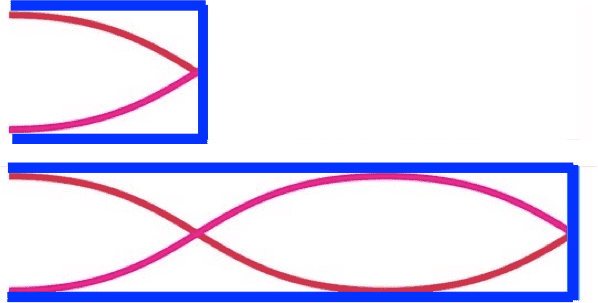# Tuning fork standing wave in a water pipe

EF17xx

## Homework StatementThe question is as follows:
The distance between the two positions of maximum loudness is x
What is the wavelength of the sound emitted by the tuning fork ?
A. x/2
B. x
C. 3x/2
D. 2x

## Homework Equations

Pipe with two ends open
λn= 2L /n

Pipe with one end open and one end closed
λn= 4L/n

## The Attempt at a Solution

At the positions of maximum loudness, there must be an antinode, but the water acts like a closed pipe so there should be a node at the surface of the water. If there is an antinode at the positions of maximum loudness the fundamental frequency would have a wavelength in distance x of λ/2. but that doesn't make sense since there should be a node so now I am confused and also don't understand how to know how many loops there should be.

#### Attachments

Homework Helper
Gold Member
The pipe will resonate at the harmonics of the tuning fork frequency. See picture below. When the pipe length is increased by x, what fraction of the wavelength is added to it?#### Attachments

EF17xx
The pipe will resonate at the harmonics of the tuning fork frequency. See picture below. When the pipe length is increased by x, what fraction of the wavelength is added to it?
View attachment 222898

I understand now, when the water surface was at the dotted line, there was still a little bit of space above in the pipe, the sound produced was the first harmonic, then when the water moved down another loud sound was heard: The second harmonic and the difference between the first and second harmonic is half a wavelength.
Therefore x=λ/2
and λ= 2x

Thanks